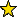# DiffAlg -- differential algebra

## Description

DiffAlg is a differential algebra package. It can compute the usual operations with polynomial differential forms and vector fields. Its main purpose is to associate algebraic objects to differential operators in the exterior algebra of differential forms.

The simplest way to load the package is with the command:

Then, one can define a linear differential 1-form, w, and the radial vector field, R, in 3-dimensional space as:

 i1 : w = newForm(2,1,1,"a") o1 = (a x + a x + a x )dx + (a x + a x + a x )dx + (a x + a x + 0 0 3 1 6 2 0 1 0 4 1 7 2 1 2 0 5 1 ------------------------------------------------------------------------ a x )dx 8 2 2 o1 : DiffAlgForm i2 : R = radial 2 o2 = x ax + x ax + x ax 0 0 1 1 2 2 o2 : DiffAlgField i3 : ring w QQ[i] o3 = ------[][a ..a ][x ..x ][dx ..dx ] 2 0 8 0 2 0 2 i + 1 o3 : PolynomialRing, 3 skew commutative variable(s) i4 : ring R QQ[i] o4 = ------[][x ..x ][ax ..ax ] 2 0 2 0 2 i + 1 o4 : PolynomialRing

All possible options to call the package can be given with the command:

loadPackage ("DiffAlg",Configuration => {"BaseRing" => aRing, "VariableName" => varSymbol, "DiffName" => difSymbol, "FieldName" => derSymbol})

where:

• aRing, a Ring, the base ring. Default QQ[i]
• varSymbol, a String, the name of the affine coordinates. Default x
• difSymbol, a String, the symbol to denote the differential of a coordinate. Default d
• derSymbol, a String, the symbol to denote the partial derivative of a coordinate. Default a

## Caveat

It is recommended to operate in low degrees and dimensions because of the computational time needed to handle the number of variables generated in every degree.

• newForm -- constructor of a differential form
• newField -- constructor of a vector field

## CertificationVersion 1.5 of this package was accepted for publication in volume 9 of The Journal of Software for Algebra and Geometry on 19 November 2018, in the article DiffAlg: a Differential algebra package. That version can be obtained from the journal or from the Macaulay2 source code repository.

## Version

This documentation describes version 1.5 of DiffAlg.

## Source code

The source code from which this documentation is derived is in the file DiffAlg.m2.

## For the programmer

The object DiffAlg is .